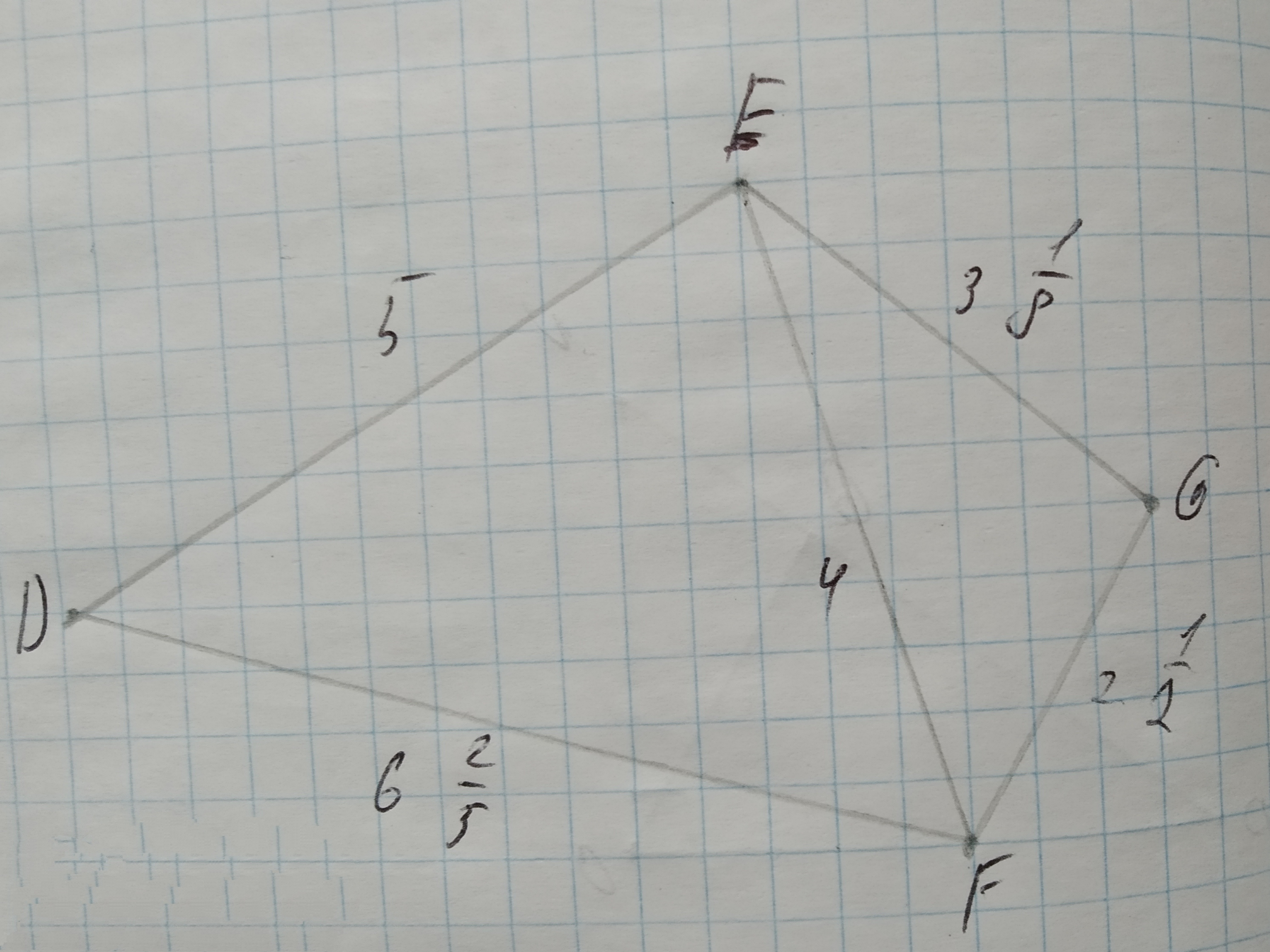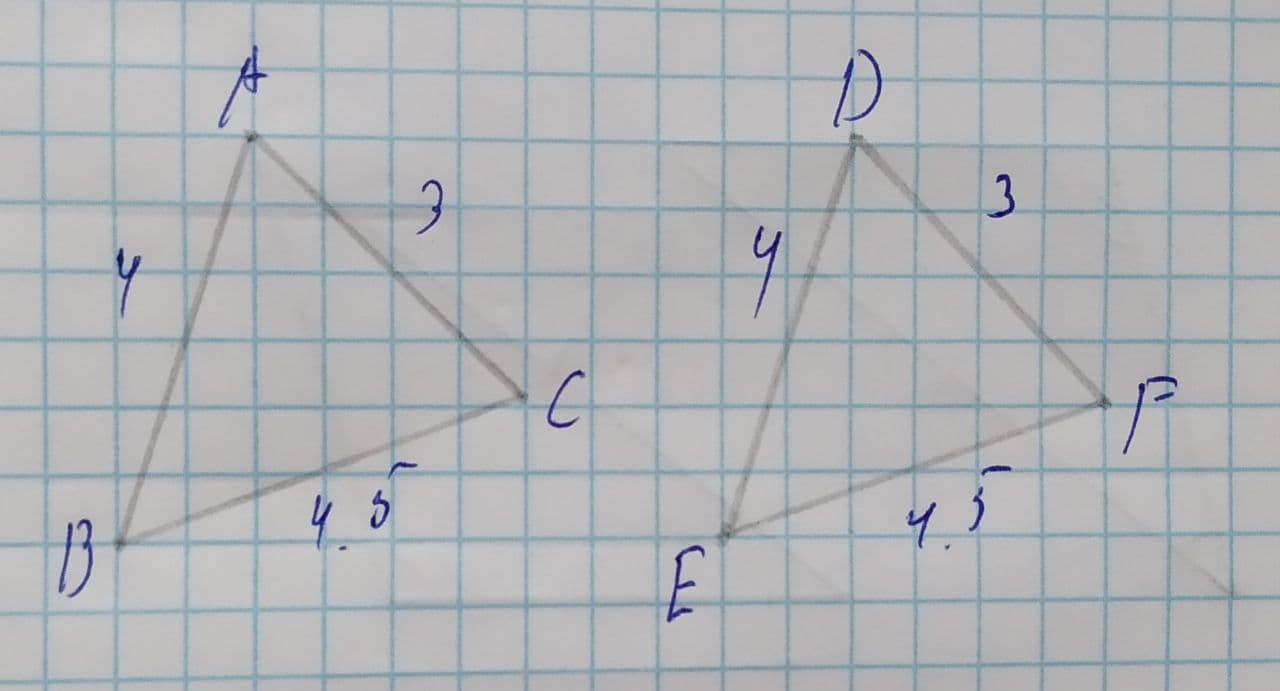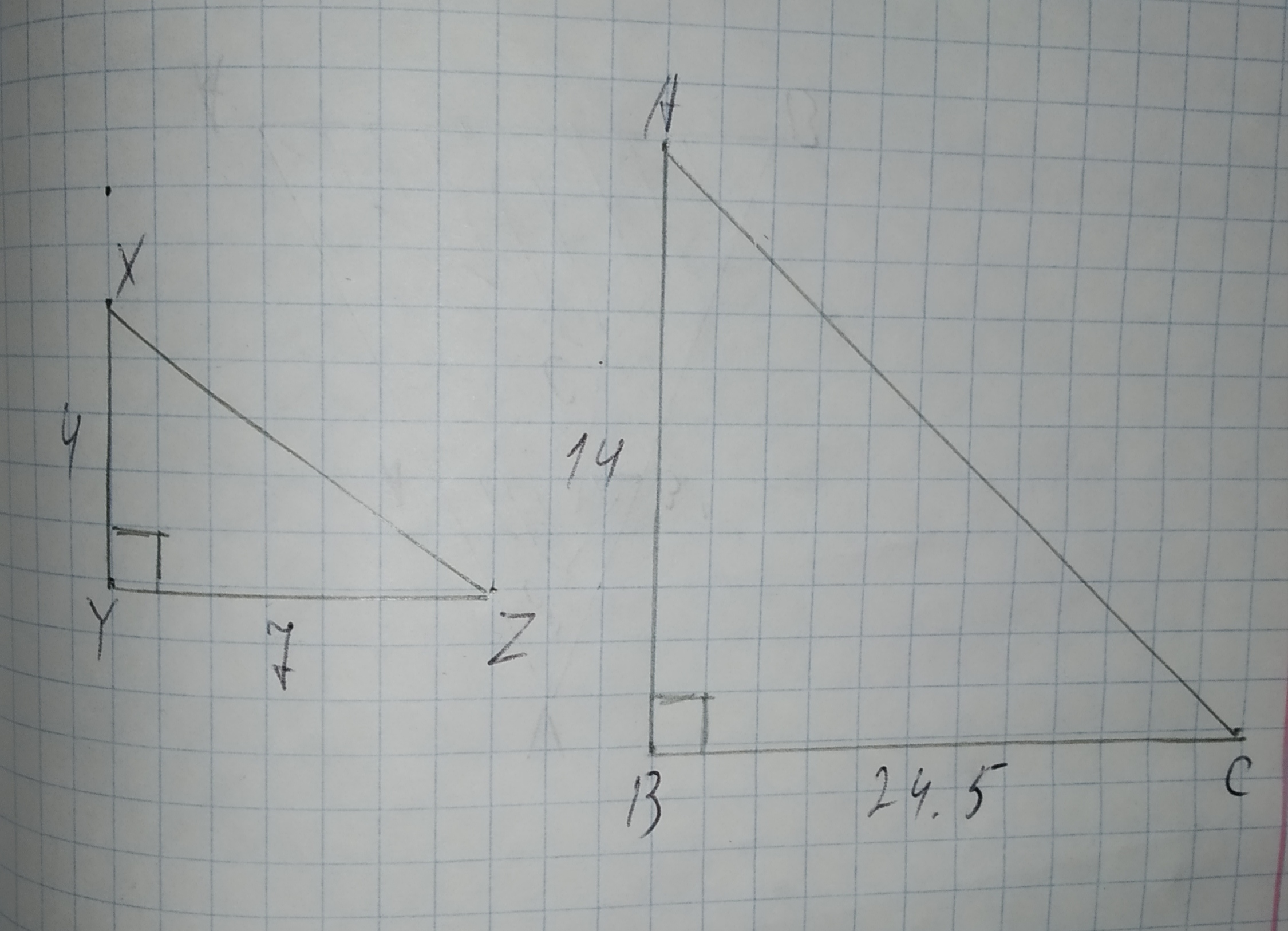Ask question

# To determine: Whether the triangle ABC and GHI are similar to each other. Given: Triangle ABC that is 75% of its corresponding side in triangle DEF. Triangle GHI that is 32% of its corresponding side in triangle DEF.# To determine: Whether the triangle ABC and GHI are similar to each other. Given: Triangle ABC that is 75% of its corresponding side in triangle DEF. Triangle GHI that is 32% of its corresponding side in triangle DEF.

Question
Similarityasked 2021-03-02
To determine: Whether the triangle ABC and GHI are similar to each other.
Given:
Triangle ABC that is 75% of its corresponding side in triangle DEF.
Triangle GHI that is 32% of its corresponding side in triangle DEF.

## Answers (1)2021-03-03
Calculation:
Since, $$\displaystyle\triangle{A}{B}{C}\sim\triangle{D}{E}{F}$$ then,
$$\displaystyle\frac{{{A}{B}}}{{{D}{E}}}=\frac{{{B}{C}}}{{{E}{F}}}$$
$$\displaystyle=\frac{{{A}{C}}}{{{D}{F}}}$$
Since, $$\displaystyle\triangle{G}{H}{I}\sim\triangle{D}{E}{F}$$ then,
$$\displaystyle\frac{{{G}{H}}}{{{D}{E}}}=\frac{{{H}{I}}}{{{E}{F}}}$$
$$\displaystyle=\frac{{{G}{I}}}{{{D}{F}}}$$
If all sides of the triangle are similar then, its angles are also similar to each other.
Then, the triangles $$\displaystyle\triangle{A}{B}{C}\sim\triangle{G}{H}{I}$$ since, the triangle $$\displaystyle\triangle{D}{E}{F}$$ is similar in both the triangle by SAS similarity.
Therefore, the triangle ABC and GHI are similar to each other by SAS similarity.

### Relevant Questionsasked 2021-03-09
To determine: The ratio of the sides of the triangles ABC and GHI.
Given:
Triangle ABC that is 75% of its corresponding side in triangle DEF.
Triangle GHI that is 32% of its corresponding side in triangle DEF.asked 2021-02-21
To prove: The similarity of $$\displaystyle\triangle{N}{W}{O}$$ with respect to $$\displaystyle\triangle{S}{W}{T}$$.
Given information: Here, we have given that NPRV is a parallelogram.asked 2021-04-16
To prove: The similarity of $$\displaystyle\triangle{B}{C}{D}$$ with respect to $$\displaystyle\triangle{F}{E}{D}$$.
Given information: Here, we have given that $$\displaystyle\overline{{{A}{C}}}\stackrel{\sim}{=}\overline{{{A}{E}}}\ {\quad\text{and}\quad}\ \angle{C}{B}{D}\stackrel{\sim}{=}\angle{E}{F}{D}$$asked 2020-10-31
Determine whether $$\displaystyle\triangle{A}{B}{C}{\quad\text{and}\quad}\triangle{D}{E}{F}$$ are similar for each set of measures. If so, identify the similarity criterion.
1.$$\displaystyle{m}\angle{A}={20},{m}\angle{C}={20},{m}\angle{D}={40},{m}\angle{F}={40}$$
2.$$\displaystyle{m}\angle{A}={20},{m}\angle{C}={4}{u},{m}\angle{D}={20},{m}\angle{F}={40}$$
3.$$\displaystyle{A}{B}={20},{B}{C}={40},{m}\angle{B}={53}$$,
$$\displaystyle{D}{E}={10},{E}{F}={20},{m}\angle{E}={53}$$asked 2021-04-15
A car initially traveling eastward turns north by traveling in a circular path at uniform speed as in the figure below. The length of the arc ABC is 235 m, and the car completes the turn in 33.0 s. (Enter only the answers in the input boxes separately given.)
(a) What is the acceleration when the car is at B located at an angle of 35.0°? Express your answer in terms of the unit vectors $$\displaystyle\hat{{{i}}}$$ and $$\displaystyle\hat{{{j}}}$$.
1. (Enter in box 1) $$\displaystyle\frac{{m}}{{s}^{{2}}}\hat{{{i}}}+{\left({E}{n}{t}{e}{r}\in{b}\otimes{2}\right)}{P}{S}{K}\frac{{m}}{{s}^{{2}}}\hat{{{j}}}$$
(b) Determine the car's average speed.
3. ( Enter in box 3) m/s
(c) Determine its average acceleration during the 33.0-s interval.
4. ( Enter in box 4) $$\displaystyle\frac{{m}}{{s}^{{2}}}\hat{{{i}}}+$$
5. ( Enter in box 5) $$\displaystyle\frac{{m}}{{s}^{{2}}}\hat{{{j}}}$$asked 2020-10-27
Given $$\displaystyle\triangle{D}{E}{F}{\quad\text{and}\quad}\triangle{E}{G}{F}$$ in the diagram below, determine if the triangles are similar. If so, write a similarity statement, and state the criterion used to support your claim.asked 2021-03-24
A man with mass 70.0 kg stands on a platform withmass 25.0 kg. He pulls on the free end of a rope thatruns over a pulley on the ceiling and has its other end fastened tothe platform. The mass of the rope and the mass of the pulley canbe neglected, and the pulley is frictionless. The rope is verticalon either side of the pulley.
a) With what force does he have to pull so that he and theplatform have an upward acceleration of $$\displaystyle{1.80}\frac{{m}}{{s}^{{2}}}$$?
b) What is the acceleration of the rope relative to him?asked 2021-04-17

State the congruence relation for $$\displaystyle\triangle{A}{B}{C}\ {\quad\text{and}\quad}\ \triangle{D}{E}{F}$$.A.SSS
B.SSA
C.AAA
D.SASasked 2021-03-26
A box weighing 70 N rests on a table. A rope tied to the box runs vertically upward over a pulley and a weight is hung from the other end. Determine the force that the table exerts on the box if the weight hanging on the other side of the pulley weighs a) 30 N b) 60 N and c) 90 Nasked 2020-12-06
To determine:Whether each pair of figures is similar and also write similarity statement and scale factor.
Given information:
Given the figure....